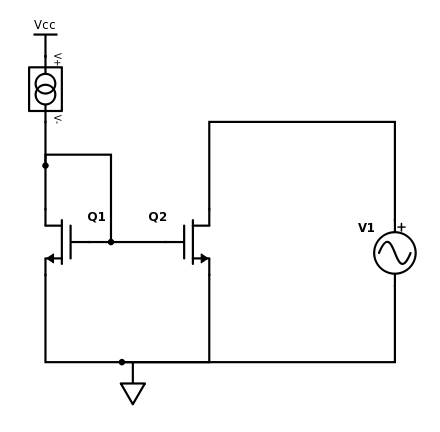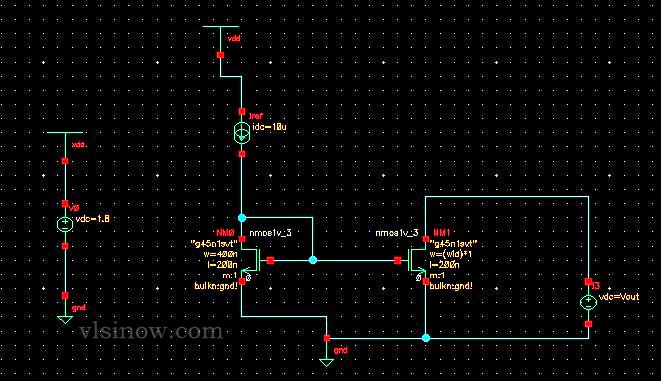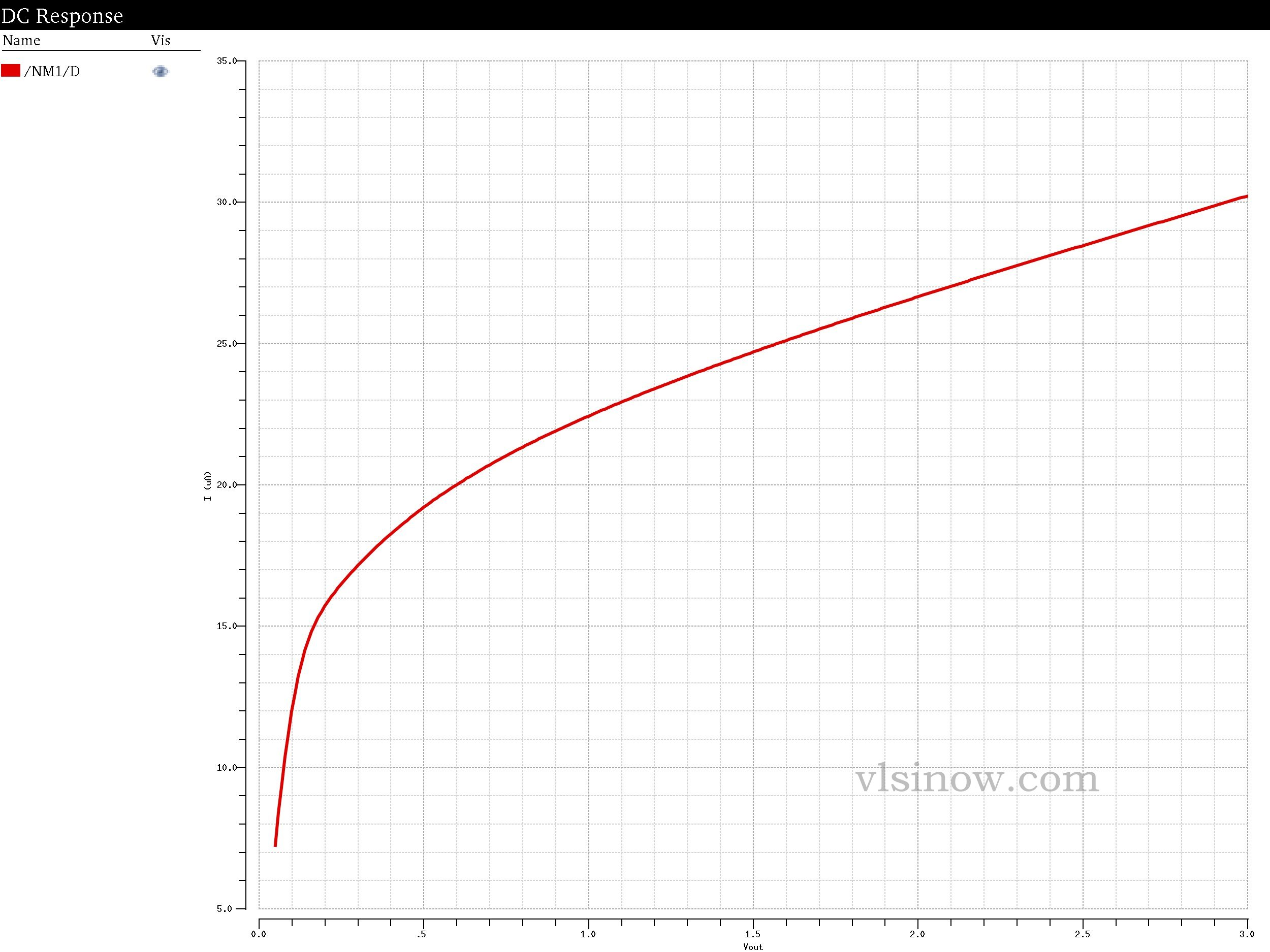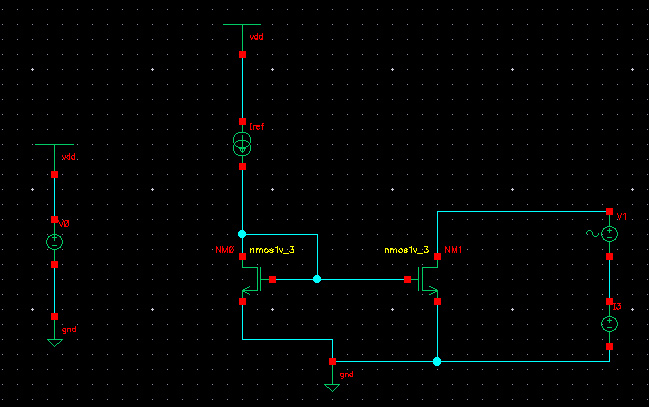# Cadence Virtuoso Simulation of Basic Current Mirror | How to Simulate Current Mirror

#### Simulate Basic Current Mirror

Current Mirror is the basic building block of analog ic design.  Here we are going to show you how to simulate basic current mirror using Cadence Virtuoso Analog Design Environment Tool.

The basic current mirror circuit diagram is given below:#### Basic Operation:

The current source will force current to the diode connected transistor Q1 which intern develop voltage VGS across gate of Q1. This VGS is given to the gate of transistor Q2. If the W/L ratios are same then it will the same current as current source. So, here we are able to mirror the current from transistor Q1 to transistor Q2.

#### Cadence Simulation of Basic Current Mirror

• Circuit Diagram• Imirror Vs Vout Plot

We plotted the output current Imirror   (Current Flowing through the NM1 transistor) with respect to output voltage Vout which is nothing but VDS of transistor NM1. Here we are using gpdk045 which is 45nm technology. Here, we are mirroring the double of reference current i.e we should get Imirror= 20uA.As we can see from the above plot because of channel length modulation the output mirrored current is not same as our input current ( current flowing through transistor NM0)  which is in this case is 10uA.  The output current increases as Vout =VDS increases. So we can conclude that basic current mirror is not a very good current mirror because the output current is not the mirrored (copied) version of input current (10uA).

Also, this graph shows that there is a minimum Vout requirement to get the output current.

• Output Resistance

To calculate the incremental output resistance we have added an ac voltage source(vsin in cadence with magnitude =1 V, Amplitude= 1uV, Frequency= 100 KHz)  vx as  shown in the below figure.  Now measure the current (ix) supplied by the voltage source .  Then the output ac resistance is given by vx/ix.Output Resistance of basic current Mirror = 135.3KOhm

• We can observe from the plot that the output resistance of basic current mirror is not that much high. It is of the order of gm/gds.
• The output  resistance of basic current mirror can be increased by using cascode current mirror.View all posts by admin →